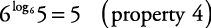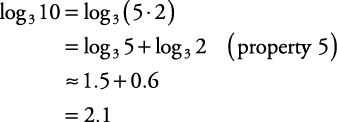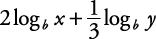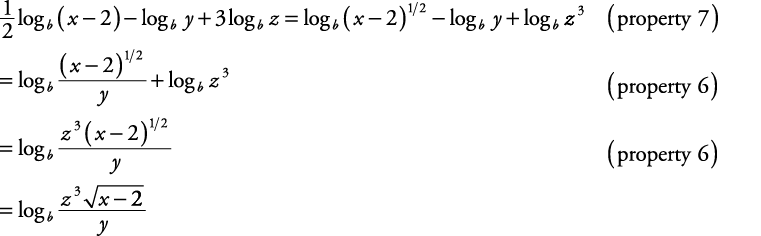## Properties of Logarithms

The properties of logarithms assume the following about the variables M, N, b, and x.1. log bb = 1

2. log b 1 = 0

3. log bb x = x

4. b logbx = x

5. log b ( MN) = log b ( M) + log b ( N)

6.Note: Don't confusewith.

To find the latter, first evaluate each log separately and then do the division.

7. log bM x = x log bM

8. If log bx = log by , then x = y.

9..

This is known as the change of base formula.

##### Example 1

Simplify each of the following expressions.

1. log 7 7

2. log 5 1

3. log 44 3

4. 6 log65

1.2.3.4.##### Example 2

If log 3 5 ≈ 1.5, log 3 3 = 1, and log 3 2 ≈ 0.6, approximate the following by using the properties of logarithms.

1. log 3 10

2.3. log 3 25

4.5. log 3 1.5

6. log 3 200

1.2.3.4.5.6.##### Example 3

Rewrite each expression as the logarithm of a single quantity.

1.2.1.2.Back to Top
A18ACD436D5A3997E3DA2573E3FD792A